# Colleges with the highest SAT scores in Maryland

Top 10 colleges in Maryland with the highest SAT scores
Looking for the colleges with the highest SAT scores in Maryland? Well you're in luck! We've compiled a national college database and have created a list of the top 10 universities with the highest SAT scores in Maryland. These are the schools whose applicants had the highest average SAT scores in Maryland. And, since these tests are meant to determine academic prowess, they are arguably the schools with the most academically proficient students. You could even say these are the best colleges in Maryland. We also include each college's ACT scores and acceptance rate so that you can see where you would have the easiest or hardest time getting in. Read on to find out more.

## Johns Hopkins University SAT scores

The average SAT score for Johns Hopkins University is 1505.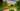The average SAT score of 1505 breaks down into:

• SAT math: 770

The average ACT score for Johns Hopkins University is 34 and their acceptance rate is 11.5%.

## University of Maryland SAT scores

The average SAT score for University of Maryland is 1380.The average SAT score of 1380 breaks down into:

• SAT math: 705

The average ACT score for University of Maryland is 31 and their acceptance rate is 44.1%.

## Saint John's College (MD) SAT scores

The average SAT score for Saint John's College (MD) is 1330.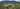The average SAT score of 1330 breaks down into:

• SAT math: 650

The average ACT score for Saint John's College (MD) is 29 and their acceptance rate is 57.6%.

## University of  Maryland Baltimore County SAT scores

The average SAT score for University of  Maryland Baltimore County is 1290.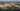The average SAT score of 1290 breaks down into:

• SAT math: 640

The average ACT score for University of  Maryland Baltimore County is 27 and their acceptance rate is 57.9%.

## United States Naval Academy SAT scores

The average SAT score for United States Naval Academy is 1260.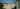The average SAT score of 1260 breaks down into:

• SAT math: 640

The average ACT score for United States Naval Academy is 29 and their acceptance rate is 8.5%.

## Loyola University Maryland SAT scores

The average SAT score for Loyola University Maryland is 1240.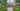The average SAT score of 1240 breaks down into:

• SAT math: 620

The average ACT score for Loyola University Maryland is 27 and their acceptance rate is 65.5%.

## Salisbury University SAT scores

The average SAT score for Salisbury University is 1220.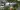The average SAT score of 1220 breaks down into:

• SAT math: 610

The average ACT score for Salisbury University is 23 and their acceptance rate is 62.2%.

## Maryland Institute College of Art SAT scores

The average SAT score for Maryland Institute College of Art is 1208.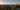The average SAT score of 1208 breaks down into:

• SAT math: 600

The average ACT score for Maryland Institute College of Art is 25 and their acceptance rate is 64.3%.

## Goucher College SAT scores

The average SAT score for Goucher College is 1200.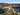The average SAT score of 1200 breaks down into:

• SAT math: 570

The average ACT score for Goucher College is 25 and their acceptance rate is 79.2%.

## Saint Mary's College of Maryland SAT scores

The average SAT score for Saint Mary's College of Maryland is 1180.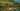The average SAT score of 1180 breaks down into:

• SAT math: 580